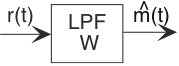6.10: Baseband Communication

Learning Objectives

• Baseband communication is the simplest form of analog communication.

We use analog communication techniques for analog message signals, like music, speech, and television. Transmission and reception of analog signals using analog results in an inherently noisy received signal (assuming the channel adds noise, which it almost certainly does).

The simplest form of analog communication is baseband communication.

Note

We use analog communication techniques for analog message signals, like music, speech, and television. Transmission and reception of analog signals using analog results in an inherently noisy received signal (assuming the channel adds noise, which it almost certainly does).

Here, the transmitted signal equals the message times a transmitter gain.

$x(t)=Gm(t)$

An example, which is somewhat out of date, is the wireline telephone system. You don't use baseband communication in wireless systems simply because low-frequency signals do not radiate well. The receiver in a baseband system can't do much more than filter the received signal to remove out-of-band noise (interference is small in wireline channels). Assuming the signal occupies a bandwidth of W Hz (the signal's spectrum extends from zero to W), the receiver applies a lowpass filter having the same bandwidth, as shown in Fig. 6.10.1 below.Fig. 6.10.1 The receiver for baseband communication systems is quite simple: a lowpass filter having the same bandwidth as the signal

We use the signal-to-noise ratio of the receiver's output $\widehat{m}(t)$ to evaluate any analog-message communication system. Assume that the channel introduces an attenuation α and white noise of spectral height $\frac{N_{0}}{2}$

The filter does not affect the signal component—we assume its gain is unity—but does filter the noise, removing frequency components above W Hz. In the filter's output, the received signal power equals α2G2 power (m) and the noise power N0W, which gives a signal-to-noise ratio of

$SNR_{baseband}=\frac{\alpha ^{2}G^{2}\: power(m)}{N_{0}W}$

The signal power power (m) will be proportional to the bandwidth W; thus, in baseband communication the signal-to-noise ratio varies only with transmitter gain and channel attenuation and noise level.

Contributor

• ContribEEOpenStax